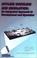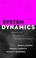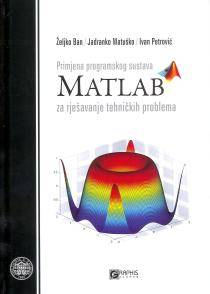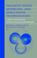### Systems Modeling and Simulation

#### Course Description

Survey of the Mathematical and graphical methods for the dynamic system description. Bond graphs. The mathematical model and parameter determination methods. Examples of the real system models. Modeling of the system with distributed parameter. Discrete event system modeling. Dynamic system simulation by block oriented languages. Numerical integration methods. Numerical optimization methods.

#### General Competencies

Introducing students to basic methods and techniques of dynamic systems modeling and simulation. Qualifying the students for dynamic system simulation using block oriented languages.

#### Learning Outcomes

1. list methods of mathematical and graphical description of dynamic systems
2. explain the dynamic changes in the system based on mathematical models
3. apply physical laws for description of the system by differential equations, difference equations, transfer functions and state space equations
4. use a mathematical description of the system for design of block diagrams, signal flow graphs and bond graphs
5. analyze the behavior of the system in steady state and during transient responses
6. identify system parameters based on dinamic system measurements
7. assemble different type of models in a single model
8. assess the credibility of models based on steady state estimates and comparisons with real system responses

#### Forms of Teaching

Lectures

Two hours lecture per week

Exams

Homeworks Mid term exame Final exam in written form Oral final exam

Consultations

After each lecture

Other

Homeworks

#### Grading Method

Continuous Assessment Exam
Type Threshold Percent of Grade Threshold Percent of Grade
Homeworks 50 % 20 % 0 % 10 %
Mid Term Exam: Written 50 % 30 % 0 %
Final Exam: Written 50 % 30 %
Final Exam: Oral 20 %
Exam: Written 50 % 40 %
Exam: Oral 50 %
##### Comment:

It is necessary to complete all homeworks for taking the final exam.

#### Week by Week Schedule

1. Topics overview, literature, organization of teaching and exams. Significance of the modeling. The process and model classification. Model equivalence. Forms of the mathematical representation of dynamic systems. Standard structures of the model representation. Transformations between standard structures. (Differential equation, transfer function, state space representation, differences equation, transfer function and state space of the discrete system, recursive equation).
2. Graphical representation of the dynamic system. (Block scheme, bond graph). Examples. Transformation of the graphics system representation into the mathematical representation of the system and vise versa. Examples of the direct methods for determining the state space representation of the system from the block scheme and bond graph representation.
3. Mathematical model determination based on the physical laws, using phenomenological and dynamic equilibrium equations.
4. Mathematical modeling of processes in the class of linear and rotary motion.
5. Mathematica modeling of the processes based on the heat and fluid dinamics.
6. Mathematical modeling of the electrical systems. Modeling of the signals defined by analytical functions.
7. Modeling the system with distributed parameters. System description by partial differential equations. Examples.
8. Midterm exam.
9. Application of the finite element method for representation of the dynamic system with distributed parameters. Examples.
10. Simulation of the dynamic system by block oriented language. Numerical integration methods.
11. Determining the dynamic system model parameters. Survey parameter identification methods, determining the model parameters by optimization process.
12. Numerical optimization methods without constrains. Bisection optimization method, golden section optimization, simplex optimization method.
13. Gradient method of optimization. Optimization with constraints. Using penalty function.
14. Discrete event system representation.
15. Final exam.

#### Study Programmes

##### University graduate
Control Engineering and Automation (profile)
Specialization Course (1. semester) (3. semester)
Electrical Engineering Systems and Technologies (profile)
Specialization Course (1. semester) (3. semester)

#### LiteratureD. J. Cloud (1998.), Applied Modeling and Simulation: An Integrated Approach to Development and Operation, McGraw-HillD. C. Karnopp, D. L. Margolis, R. C. Rosenberg (2000.), System Dynamics: Modeling and Simulation of Mechatronic Systems, John Wiley & sons, IncŽ. Ban, J. Matuško, I. Petrović (2010.), Primjena programskog sustava Matlab za rješavanje tehničkih problema, Graphis, ZagrebH. S. Sarjoughian, F. E. Cellier (2001.), Discrete Event Modeling and Simulation Technologies: A Tapestry of Systems and AI-Based Theories and Methodologies, Springer

#### General

ID 34376
Winter semester
4 ECTS
L1 English Level
L1 e-Learning
30 Lectures
0 Exercises
0 Laboratory exercises
0 Project laboratory

87,5 Excellent
75 Very Good
62,5 Good
50 Acceptable